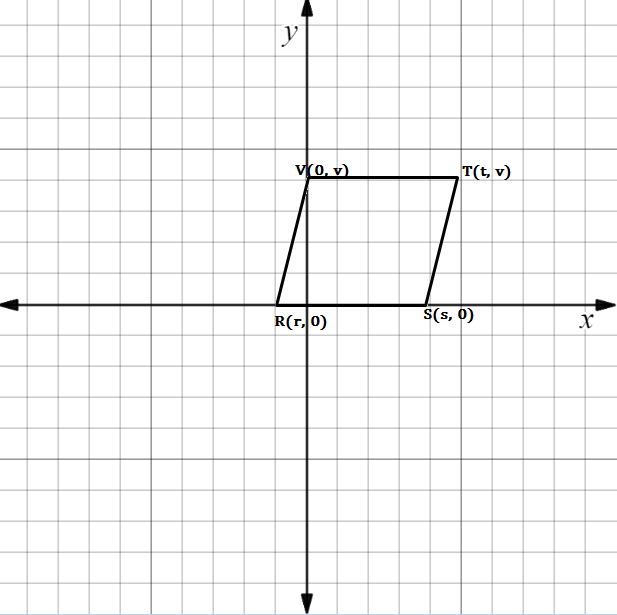Chapter 10.CT, Problem 15CT### Elementary Geometry for College St...

6th Edition
Daniel C. Alexander + 1 other
ISBN: 9781285195698

#### Solutions

Chapter
Section### Elementary Geometry for College St...

6th Edition
Daniel C. Alexander + 1 other
ISBN: 9781285195698
Textbook Problem
1 views

# In the figure, we see that m R S - = m V T - = 0 . Find the equation that relates r ,   s ,   a n d   t if it is known that R S T V is a parallelogram.                                                                                                 _To determine

To find:

The equation that relates r, s, and t if it is known that RSTV is a parallelogram.

Explanation

Given figure is the parallelogram RSTV.

Here the slope of RS=VT=0

That is mRS¯=mVT¯=0

And the slope of VR= the slope of TS

That is mVR¯=mST¯

Formula for slope of the line is m=y2-y1x2-x1

Slope of VR:

x1, y1=(0, v)

x2, y2=(r,0)

Substituting the above values in the formula,

mVR¯=0-vr-0

mVR¯=

### Still sussing out bartleby?

Check out a sample textbook solution.

See a sample solution

#### The Solution to Your Study Problems

Bartleby provides explanations to thousands of textbook problems written by our experts, many with advanced degrees!

Get Started